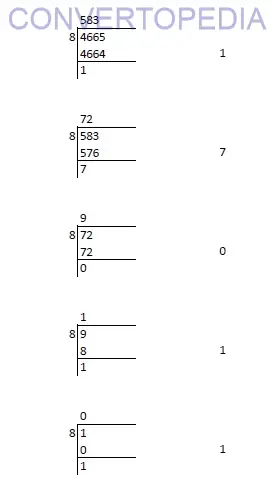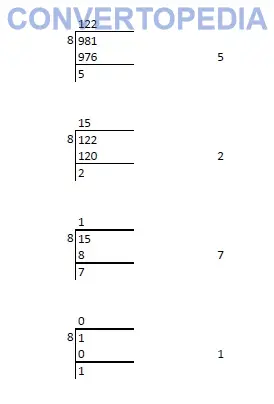# How to convert Decimal number to octal

## Decimal Number

Whole number, a decimal point and a fractional value combines to form a decimal number. The decimal point separates the whole number part from the fractional part of the number. Each digit of a decimal number can be any number from 0 to 9. Any value less than 1 is written to the right of decimal point. Decimal numbers are also known as base-10 number or counting numbers. Place value of decimal number varies as the whole number powers of 10 starting from the left of decimal point. Similarly, the place value of digits left to decimal point varies as the division of power of tens.

## Octal Number

Octal numbers use digits from 0-7 only. It is known as base-8 number. The place value of each digits of an octal number varies as the whole number powers of 8 starting from the right (Least Significant Digit). The first single digit number in octal system is 0 and the last is 7. Similarly, the first two digit octal number is 10 and the last is 77 and so on. Octal number system was widely used in early computers.

## How to convert decimal to octal:

To convert any decimal number to octal follow the below steps:

### Step 1:

Divide the number by 8 and note down the quotient and reminder. Let it be reminder-1 and quotient-1 respectively.

### Step 2:

Again divide the quotient-1 by 8 and note down the quotient and remainder as reminder-2 and quotient-2.

### Step 3:

Repeat Step-1 and Step-2 until the quotient becomes zero.

### Step 4:

Assume you have done the division ‘n’ times until you got a quotient of 0. From the first reminder, write down all the reminders from right to left.

## Example for Decimal number to octal conversion

### Example-1

#### Convert 466510 to octalHence, 466510 = 110718

### Example-2

#### Convert 98110 to octalHence, 98110 = 17258

## Decimal to octal conversion table

Decimal NumberOctal Number
1 1
2 2
3 3
4 4
5 5
6 6
7 7
8 10
9 11
1012
1113
1214
1315
1416
1517
1620
5062
100144